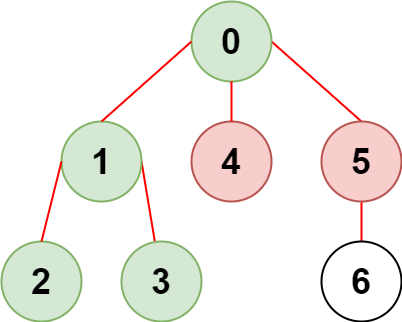##### Welcome to Subscribe On Youtube

Formatted question description: https://leetcode.ca/all/2368.html

# 2368. Reachable Nodes With Restrictions

• Difficulty: Medium.
• Related Topics: Array, Hash Table, Tree, Depth-First Search, Breadth-First Search, Graph.
• Similar Questions: Open the Lock, Minimum Jumps to Reach Home.

## Problem

There is an undirected tree with n nodes labeled from 0 to n - 1 and n - 1 edges.

You are given a 2D integer array edges of length n - 1 where edges[i] = [ai, bi] indicates that there is an edge between nodes ai and bi in the tree. You are also given an integer array restricted which represents restricted nodes.

Return the **maximum number of nodes you can reach from node 0 without visiting a restricted node.**

Note that node 0 will not be a restricted node.

Example 1:Input: n = 7, edges = [[0,1],[1,2],[3,1],[4,0],[0,5],[5,6]], restricted = [4,5]
Output: 4
Explanation: The diagram above shows the tree.
We have that [0,1,2,3] are the only nodes that can be reached from node 0 without visiting a restricted node.


Example 2:Input: n = 7, edges = [[0,1],[0,2],[0,5],[0,4],[3,2],[6,5]], restricted = [4,2,1]
Output: 3
Explanation: The diagram above shows the tree.
We have that [0,5,6] are the only nodes that can be reached from node 0 without visiting a restricted node.


Constraints:

• 2 <= n <= 105

• edges.length == n - 1

• edges[i].length == 2

• 0 <= ai, bi < n

• ai != bi

• edges represents a valid tree.

• 1 <= restricted.length < n

• 1 <= restricted[i] < n

• All the values of restricted are unique.

## Solution (Java, C++, Python)

• class Solution {
public int reachableNodes(int n, int[][] edges, int[] restricted) {
List<Integer>[] graph = new ArrayList[n];
for (int i = 0; i < n; i++) {
graph[i] = new ArrayList<>();
}
for (int[] edge : edges) {
int src = edge;
int dest = edge;
}
Queue<Integer> q = new ArrayDeque<>();
boolean[] visited = new boolean[n];
q.offer(0);
visited = true;
for (int node : restricted) {
visited[node] = true;
}
int ans = 0;
while (!q.isEmpty()) {
int vertex = q.poll();
ans++;
for (int neighbour : graph[vertex]) {
if (!visited[neighbour]) {
q.offer(neighbour);
visited[neighbour] = true;
}
}
}
return ans;
}
}

############

class Solution {
private List<Integer>[] g;
private boolean[] vis;
private int ans;

public int reachableNodes(int n, int[][] edges, int[] restricted) {
g = new List[n];
Arrays.setAll(g, k -> new ArrayList<>());
vis = new boolean[n];
for (int v : restricted) {
vis[v] = true;
}
for (int[] e : edges) {
int a = e, b = e;
}

ans = 0;
dfs(0);
return ans;
}

private void dfs(int u) {
if (vis[u]) {
return;
}
++ans;
vis[u] = true;
for (int v : g[u]) {
dfs(v);
}
}
}

• class Solution:
def reachableNodes(
self, n: int, edges: List[List[int]], restricted: List[int]
) -> int:
g = defaultdict(list)
vis = [False] * n
for v in restricted:
vis[v] = True
for a, b in edges:
g[a].append(b)
g[b].append(a)

def dfs(u):
nonlocal ans
if vis[u]:
return
ans += 1
vis[u] = True
for v in g[u]:
dfs(v)

ans = 0
dfs(0)
return ans

############

# 2368. Reachable Nodes With Restrictions
# https://leetcode.com/problems/reachable-nodes-with-restrictions/

class Solution:
def reachableNodes(self, n: int, edges: List[List[int]], restricted: List[int]) -> int:
graph = defaultdict(list)
visited = [False] * n
blocked = set(restricted)

for x, y in edges:
graph[x].append(y)
graph[y].append(x)

res = 0

def dfs(node):
nonlocal res

visited[node] = True

res = 1

for nei in graph[node]:
if nei not in blocked and not visited[nei]:
res += dfs(nei)

return res

return dfs(0)


• class Solution {
public:
int ans;

int reachableNodes(int n, vector<vector<int>>& edges, vector<int>& restricted) {
vector<vector<int>> g(n);
for (auto& e : edges) {
int a = e, b = e;
g[a].push_back(b);
g[b].push_back(a);
}
vector<bool> vis(n);
for (int v : restricted) vis[v] = true;
ans = 0;
dfs(0, g, vis);
return ans;
}

void dfs(int u, vector<vector<int>>& g, vector<bool>& vis) {
if (vis[u]) return;
vis[u] = true;
++ans;
for (int v : g[u]) dfs(v, g, vis);
}
};

• func reachableNodes(n int, edges [][]int, restricted []int) int {
g := make([][]int, n)
for _, e := range edges {
a, b := e, e
g[a] = append(g[a], b)
g[b] = append(g[b], a)
}
vis := make([]bool, n)
for _, v := range restricted {
vis[v] = true
}
ans := 0
var dfs func(u int)
dfs = func(u int) {
if vis[u] {
return
}
vis[u] = true
ans++
for _, v := range g[u] {
dfs(v)
}
}
dfs(0)
return ans
}

• function reachableNodes(
n: number,
edges: number[][],
restricted: number[],
): number {
let res = 0;
const vis = new Array(n).fill(false);
const map = new Map<number, number[]>();
for (const [start, end] of edges) {
map.set(start, [...(map.get(start) ?? []), end]);
map.set(end, [...(map.get(end) ?? []), start]);
}
const dfs = (cur: number) => {
if (restricted.includes(cur) || vis[cur]) {
return;
}
res++;
vis[cur] = true;
for (const item of map.get(cur) ?? []) {
dfs(item);
}
};
dfs(0);

return res;
}



Explain:

nope.

Complexity:

• Time complexity : O(n).
• Space complexity : O(n).